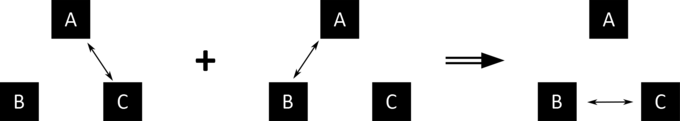Zeroth Law of Thermodynamics - Thermal Equilibrium

Zeroth Law of Thermodynamics:

Before the zeroth law of thermodynamics was put forward, there had previously been in place 3 laws of Thermodynamics. They are:

• 1st Law of Thermodynamics
• 2nd Law of Thermodynamics
• 3rd Law of Thermodynamics

Our previous article states the law HERE

Scientists came to a realization that these 3 sets of laws aren't exactly complete as there is a need for a law that will introduce a formal definition of temperature. Because of the nature and importance of this law, it needed to supersede the three existing laws. Since these three laws are already widely known by their numbers, a kind of numbering confusion came up. The alternative of calling this new law the Fourth Law woundn't sound very right because of it's importance. It was Ralph Fowler, a scientist that called the new law the “Zeroth Law" which had become popular to this very day.

The Zeroth Law of Thermodynamics states that if two separate bodies are in thermal equilibrium with another body, then they are also in equilibrium with each other

To better understand what the zeroth law meant, we need to know what thermal equilibrium is;

Two bodies A and B are said to be in thermal equilibrium if each body can transfer heat to each other when placed closed together but ended up NOT transferring any heat to each other. This will mean that both bodies are having exactly the same temperature.

Now, according to the zeroth law, assuming we have three bodies namely X, Y and Z:

if X = Y and Z = Y, then X = Z

It can be further said that if X and Y are in thermal equilibrium and Z and Y are in thermal equilibrium, therefore, X and Z must be in thermal equilibrium too.

So we can see that according to the zeroth law, Temperature is a quantity worth measuring.

When we put a pot of water on a cooking gas, we notice the transfer of heat from the gas to water and the water begins to get warm. As heat transfer continues, the water becomes warmer and eventually becomes hot. A point will be reached when the water starts to boil (boiling point = 100OC for pure water). Thermal equilibrium is reached because at this temperature, the water cannot become hotter, but instead, it evaporates as water vapour.

Although, Temperature is not directly mentioned in the zeroth law, the law actually points us towards the existence of temperature as a quantity. It can therefore be stated that the quantity that is always the same for all systems in thermal equilibrium with one another is TEMPERATURE.Image Credit: S3-us-west-2.amazonawsAlfred Ajibola is a Medical Biochemist, a passionate Academician with over 7 years of experience, a Versatile Writer, a Web Developer, a Cisco Certified Network Associate and a Cisco CyberOps Associate.